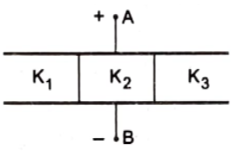The space between the plates of a parallel-plate capacitor of capacitance C is filled with three dielectric slabs of identical size as shown in figure. If the dielectric constants of the three slabs areK1, K2  and  K3,  then find the new capacitance

# The space between the plates of a parallel-plate capacitor of capacitance C is filled with three dielectric slabs of identical size as shown in figure. If the dielectric constants of the three slabs are  then find the new capacitance1. A

${\mathrm{C}}_{\mathrm{eq}}=\left({\mathrm{K}}_{1}+{\mathrm{K}}_{2}+{\mathrm{K}}_{3}\right)\frac{\mathrm{C}}{3}$

2. B

${\mathrm{C}}_{\mathrm{eq}}=\left({\mathrm{K}}_{1}+{\mathrm{K}}_{2}+{\mathrm{K}}_{3}\right)\frac{2\mathrm{C}}{3}$

3. C

${\mathrm{C}}_{\mathrm{eq}}=\left({\mathrm{K}}_{1}+{\mathrm{K}}_{2}+{\mathrm{K}}_{3}\right)\frac{3\mathrm{C}}{3}$

4. D

${\mathrm{C}}_{\mathrm{eq}}=\left({\mathrm{K}}_{1}+{\mathrm{K}}_{2}+{\mathrm{K}}_{3}\right)\frac{4\mathrm{C}}{3}$

Register to Get Free Mock Test and Study Material

+91

Verify OTP Code (required)

### Solution:

Consider each one third of the assembly as a separate capacitor. The three positive plates are connected together at point A and the three negative plates are connected together at point B. Thus, the three capacitors are joined in parallel. As the plate area is one third of the original for each part, the capacitances of these parts will be ${\mathrm{K}}_{1}\mathrm{C}/3,\text{\hspace{0.17em}\hspace{0.17em}}{\mathrm{K}}_{2}\mathrm{C}/3$  and ${\mathrm{K}}_{3}\mathrm{C}/3$  . The equivalent capacitance is, therefore,  ${\mathrm{C}}_{\mathrm{eq}}=\left({\mathrm{K}}_{1}+{\mathrm{K}}_{2}+{\mathrm{K}}_{3}\right)\frac{\mathrm{C}}{3}$Register to Get Free Mock Test and Study Material

+91

Verify OTP Code (required)